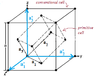# Interplanar Spacing: Calculating Distance between (200) Planes

• aaaa202

#### aaaa202

The interplanar spacing between planes labeled by miller indices (hkl) is given by:
d=2$\pi$/lGl
where G is the reciprocal lattice vector:
G=hb1+kb2+kb3
Somehow I can't get this formula to work. I wonna calculate the distance between (200) planes. This should be a/2, where a is the lattice constant. But (look it up) b1=2$\pi$/a (-x + y +z), where x, y and z are unit vectors. And so G= hb1 = 2$\pi$/a (-x + y +z) and thus lGl = h2$\pi$/a * √3
=>
d= a/(2√3)
But this can't be right. I have no idea why I get that factor of √3, because I checked everything. Where did I make a silly mistake?

From your expression for b1, it appears that you are working with a face-centered-cubic lattice (in real space) and that you have chosen reciprocal lattice vectors that are reciprocal to a set of primitive lattice vectors corresponding to a primitive cell. However, for fcc and bcc lattices the miller indices are often chosen to correspond to a conventional cell.
For information on primitive and conventional cells: http://www-personal.umich.edu/~sunkai/teaching/Winter_2013/third_lecture.pdf

The unprimed vectors (a1, a2, a3) in the diagram that I attached show lattice vectors in real space that correspond to a primitive cell of the lattice. The primed vectors correspond to a conventional cell.

So, the Miller indices (2, 0, 0) probably correspond to the conventional cell. If so and you want to use d=2$\pi$/lGl, you will need to find the reciprocal vector b'1 that is reciprocal to the conventional cell lattice vectors (a'1, a'2, a'3).

#### Attachments

•cells.png
8.8 KB · Views: 652
Last edited: